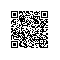# L1-019. 谁先倒

L1-019. 谁先倒

1 1
6
8 10 9 12
5 10 5 10
3 8 5 12
12 18 1 13
4 16 12 15
15 1 1 16

A
1

#include <iostream>
using namespace std;
int main()
{
int a, b;
int flag = 0;
int count;  //没倒的人的杯数
cin >> a >> b;
int n;
cin >> n;
int aa = 0, bb = 0;
for(int i = 0; i < n; i++) {
int j, jj, y, yy;
cin >> j >> jj >> y >> yy;  //甲喊 甲划 乙喊 乙划
if(jj == j + y && yy != j + y) {    //甲输
aa++;
} else if(yy == j + y && jj != j + y) {
bb++;
}
if(aa > a) {
flag = 1;
count = bb;
break;

}
if(bb > b) {
flag = 2;
count = aa;
break;
}
}
if(flag == 1) {
cout << "A" <<endl;
cout <<count;
} else {
cout << "B" <<endl;
cout <<count;
}
return 0;
}使用钉钉扫一扫加入圈子
+ 订阅